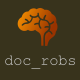# Creating objects in Pico-8 using tables to imitate classesAfter a while, it becomes necessary to use objects in your program.  That is, you create a class of object (let’s say a bullet) which can control its own update and draw routines and keeps track of its location, interactions and status.

Note: I have a YouTube tutorial that explains all of this here.

This is a very powerful concept in programming – instead of creating separate variables for every bullet in a game, you define an class and then simply keep track of all the objects that are created (or instantiated) from this class.  It’s a tricky concept, but this is roughly how it works:A class is created with variables (in this case, `owner`, `x`,` y` and `velocity`) and some functions such as `move()`,` hit()` and `get_pos()`.  We can then create a new instance of that class (an object).  Each object (there are 4 in the diagram) has a unique set of variables (as defined by the class) and has access to the functions of the class.  So every bullet can `move()` or `hit()` etc.

In Pico-8 it is possible to produce this style of programming quite easily using tables.

A table in Pico-8 is a composite data structure which contains a list of keys and values.  They are constructed using a comma separated list between curly brackets like this:

`my_table={a=2,b=3,c="hello",d=velocity}`

What is important is that, in Pico-8, tables do not need to contain the same data types, so we can combine integers, strings and, importantly, even functions.

To create a class in Pico-8 we first need to declare an empty table to contain all the objects for our class.  In this instance, we will use bullets.

```function _init()
bullets={}
end```

We then need to create a function that will add object to this table.  Effectively, what our function will do is to create an instance of our class and add it to the table.

```function add_new_bullet()
end
```

This function makes use of the `add()` function to add a table (`{}`) to the bullets table.  Obviously, this is not going to be effective and it will require more detail!

Firstly, we can expand the empty table to set some variables like this

```function add_new_bullet()
x=63,
y=63,
dx=2,
dy=2,
life=10
})
end
```

This adds some variables for position, speed and lifespan.  Note that each is separated by a comma as it is a separate mapping in the table.

Now, the interesting part: we want the class to take care of its own functions.  We can do this by adding a new mapping where we map an entire function to a variable.

```function add_new_bullet()
x=63,
y=63,
dx=2,
dy=2,
life=10
draw=function(self)
pset(self.x,self.y,8)
end
})
end
```

Here the variable draw contains a function that will draw a pixel at the x and y location of the bullet.  Note that, in order to reference the correct variables we need the self. identifier in front of each variable and we also make sure the function calls itself in order to act on the correct object.

We can also add an update function like this:

```function add_new_bullet()
x=63,
y=63,
dx=2,
dy=2,
life=10,
draw=function(self)
pset(self.x,self.y,8)
end,
update=function(self)
self.x+=self.dx
self.y+=self.dy

self.life-=1
if self.life<0 then
del(dust,self)
end
end
})
end
```

Here we have changed the x and y value by the speed to move the object.  We have also added a section to take care of the age of the object so that we can remove it from the table after a certain period of time.  If we don’t do this, we will end up with too many objects in the game and the game will slow down.

Now, we have created our class and the function that will instantiate a new object for it.  So, in our update routine we can add a new object by calling the `add_new_bullet()` function.

To update all the bullets we simply put this for loop in the `_update()` function:

```for b in all(bullets) do
b:update()
end```

and to draw it we put

```for b in all(bullets) do
b:draw()
end```

in the `_draw()` function.

The final thing to mention is that it is possible, in your `add_new_bullet()` function to pass variables to the new object.  As an example, here is the function modified to pass an x and y value to the new object (note, I use `_x` and `_y` to distinguish between the passed values and the objects own values.

```function add_new_bullet(_x,_y)
x=_x,
y=_y,
dx=2,
dy=2,
life=10,
draw=function(self)
pset(self.x,self.y,8)
end,
update=function(self)
self.x+=self.dx
self.y+=self.dy

self.life-=1
if self.life<0 then
del(dust,self)
end
end
})
end```

And that’s it!  It’s a remarkably simple method, but it can be used for all sorts of effects.

Happy programming!

### You May Also Like

1.Ian Wood says:

I’ve been using this method for my project where an autogun tracks an enemy and fires on them, but I have reached a dead end because my bullet class can only track one enemy class at a time.

this is my bullet update function –

update = function(self)

self.dx=en.x-self.x –This is where I’m stumped
self.dy=en.y-self.y –How to code for en[1,2,3,4,5….]

d =sqrt(self.dx*self.dx+self.dy*self.dy)
del(bullet,self)
end
self.x+=self.dx/d
self.y+=self.dy/d
end
if d<1 then
del(bullet,self)
end
end,
For the life of me I can't get the autogun to track all instances of the enemy, only the first or last or specified number. As you can see, the enemy are a table of tables the same as the bullets. Any thoughts?

Cheers

1.Doc Robs says:

Hi,

Looking at the code, you will need to pass it a parameter which represents which enemy to track (you’ve hard coded it to 1 by the looks of things. If you can caluclate which enemy is nearest, for example, you could store that value and send it to the update routine each turn.